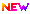High Impact Factor : 4.396| Submit Manuscript Online|

## Modification of Setup for Major Losses in Pipes to Determine the Exact Value of Friction Factor

### Author(s):

Shubham Ghadge , GCOERC, Nashik; Harshal Barbade, GCOERC, Nashik; Abhishek Mhaske, GCOERC, Nashik; Chetan Malode, GCOERC, Nashik; Pritam Kudale, GCOERC, Nashik

### Keywords:

Darcy Friction Factor, Major Losses in Pipes

### Abstract

in this research paper we determine the exact value of friction factor through pipes for turbulent flow. Friction factor conceptually defined as the ratio of two forces acting, respectively, perpendicular & parallel to an interface between two bodies under relative motion or impending relative motion, this dimensionless quantity turns out to be convenient for depicting the relative ease with which the materials slide over each other under particular circumstances. This includes applications of various equations such as Darcy-Weisbach equation, Swamee-Jain equation, Colebrook equation, Reynolds equation. This formulae provide a base for statistical evaluation of exact friction factor for smooth as well as rough pipes. The Darcy friction factor for turbulent flow must be solved by using Colebrook equation by using iteration method. By using iteration method new equations are evolved. These equations can be further developed by using Blasius, Swamee-Jain & Haaland equations. All the three equations are applicable but the Blasius equation cannot be used for rough pipes whereas the Swami-Jain & Haaland equations are applicable for rough pipes and give good results in order to determine the friction factor for rough pipes.

### Other Details

Paper ID: IJSRDV5I21079
Published in: Volume : 5, Issue : 2
Publication Date: 01/05/2017
Page(s): 1135-1137